# Problem 53930. Easy Sequences 65: Fractional Part of Square Roots

The fractional part function of a positive real number r, denoted as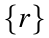, is defined as: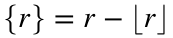, where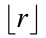, is the 'floor' of r. Thus,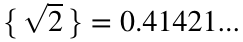,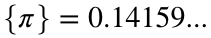and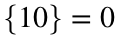.
Given a positive integer n, create the function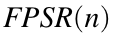, that evaluates the following summation:For example for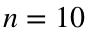:Please present the function output rounded-off to nearest integer. Therefore, for, the function should return 3.
HINT: There are efficient algorithms to calculate fractional part of square root.

### Solution Stats

55.56% Correct | 44.44% Incorrect
Last Solution submitted on Sep 11, 2023

### Community Treasure Hunt

Find the treasures in MATLAB Central and discover how the community can help you!

Start Hunting!